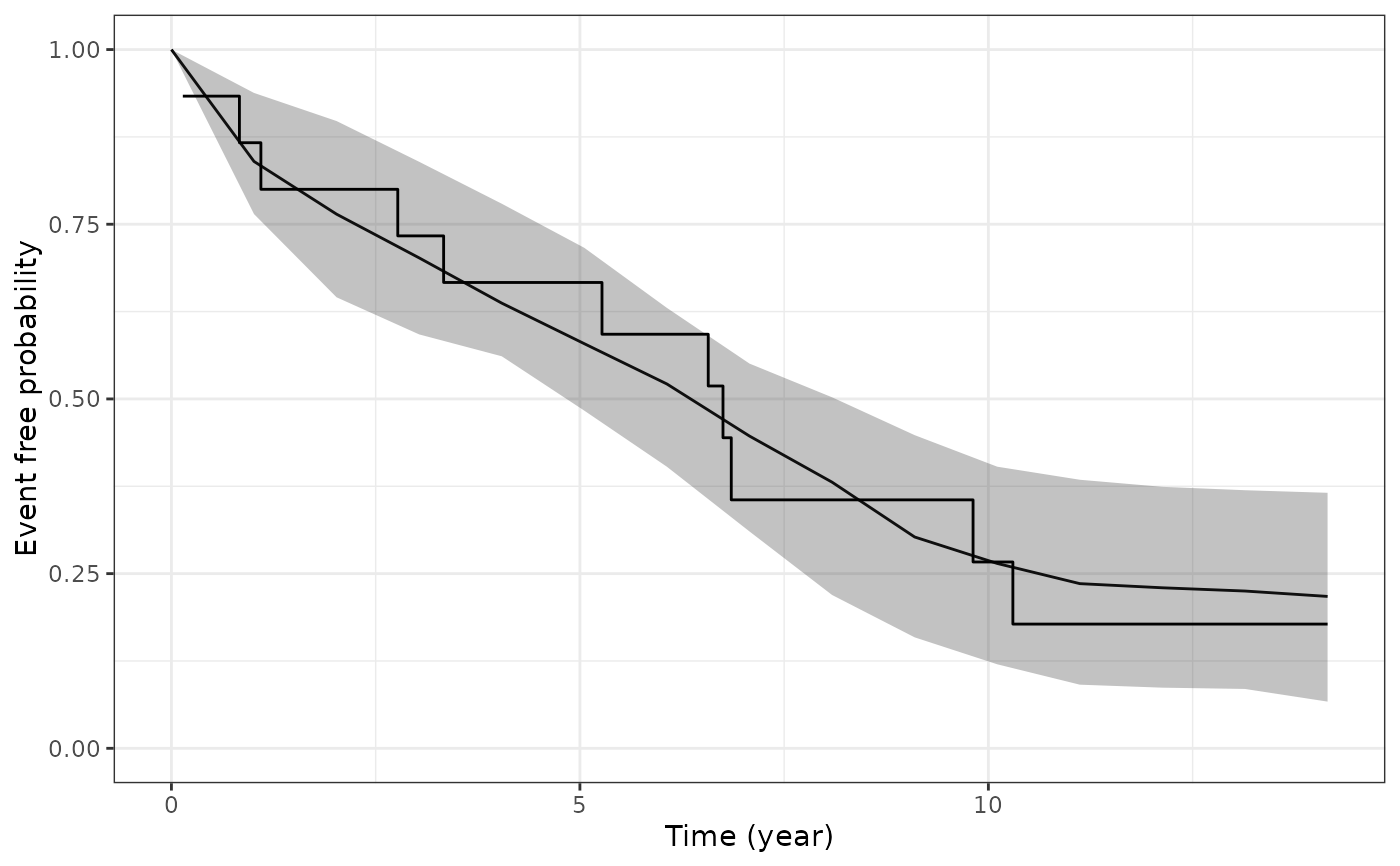This function plots the estimated marginal survival function based on draws from the posterior predictive distribution of the fitted joint model, and then overlays the Kaplan-Meier curve based on the observed data.

ps_check(
object,
check = "survival",
limits = c("ci", "none"),
draws = NULL,
seed = NULL,
xlab = NULL,
ylab = NULL,
ci_geom_args = NULL,
...
)

## Arguments

object A fitted model object returned by the stan_jm modelling function. See stanreg-objects. The type of plot to show. Currently only "survival" is allowed, which compares the estimated marginal survival function under the joint model to the estimated Kaplan-Meier curve based on the observed data. A quoted character string specifying the type of limits to include in the plot. Can be one of: "ci" for the Bayesian posterior uncertainty interval (often known as a credible interval); or "none" for no interval limits. An integer indicating the number of MCMC draws to use to to estimate the survival function. The default and maximum number of draws is the size of the posterior sample. An optional seed to use. An optional axis label passed to labs. Optional arguments passed to geom_ribbon and used to control features of the plotted interval limits. They should be supplied as a named list. Optional arguments passed to geom_line and used to control features of the plotted trajectory.

## Value

A ggplot object that can be further customized using the ggplot2 package.

posterior_survfit for the estimated marginal or subject-specific survival function based on draws of the model parameters from the posterior distribution, posterior_predict for drawing from the posterior predictive distribution for the longitudinal submodel, and pp_check for graphical checks of the longitudinal submodel.

## Examples

# \donttest{
if (!exists("example_jm")) example(example_jm)
# Compare estimated survival function to Kaplan-Meier curve
ps <- ps_check(example_jm)
ps +
ggplot2::scale_color_manual(values = c("red", "black")) + # change colors
ggplot2::scale_size_manual(values = c(0.5, 3)) + # change line sizes
ggplot2::scale_fill_manual(values = c(NA, NA)) # remove fill# }